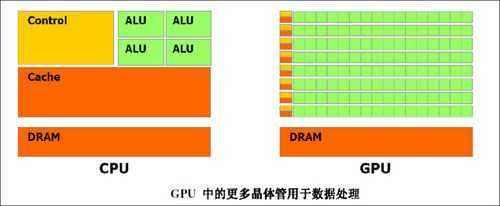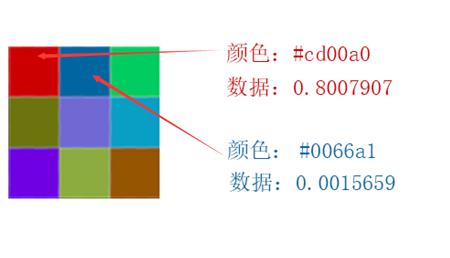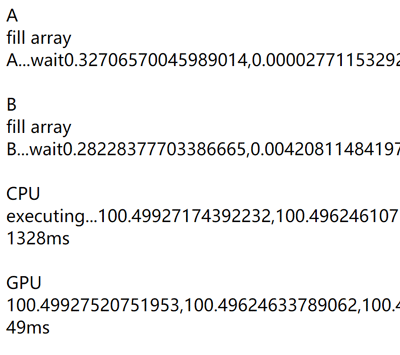# 通过 GPU 加速浏览器浮点数计算能力CPU VS GPUGPU 面临的情况则不同，3D 空间顶点变换是一种类型完全相同的计算，只不过数量较大而已，完全可以打造出多条与任务匹配的 “临时流水线” 并行工作，分担这些工作。GLSL

GLSL 是一种可编程着色器语言，通过它 ，我们可以将大量的 GPU 计算单元组成临时的流水线。 GLSL 原生支持向量、矩阵计算，可以方便的在编程中完成空间变形。 WebGL 中也需要用到 GLSL ，源码一般存在 JS 文件中，使用时被编译成着色器对象。受篇幅限制，关于更多 GLSL 的信息在 GLSL-Card 中文手册

### Step 1. 编写 GLSL 版的计算程序

JS 版本:

``let someFloat = Math.pow(0.123456789,2)``

GLSL 版本:

``````... ...
attribute float a_somefloat;
void main() {
... ...
flost somefloat = pow(a_somefloat,2.0);
... ...
}``````

``````let canvas = document.createElement("canvas"); // 创建 canvas 对象
canvas.width = 256;
canvas.height = 256;
let gl = getContext("webgl");//获取 GL 上下文``````

``````... ... //编译着色器对象
gl.bindBuffer(gl.ARRAY_BUFFER, bufferObject);
... ...
let a_somefloat = gl.getAttribLocation(currentProgram, 'a_somefloat'); //读取 GLSL 变量位置
gl.vertexAttribPointer(a_somefloat, 1, gl.FLOAT, false, 0, 0); // 发送数据
... ...``````

### Step 2. GLSL 分割计算结果

``````0.123456789/(1/256) =  31.604937984

``````0.0023630389999999973/(1/256/256) = 154.86412390399983

``````0.000013185484374997336/(1/256/256/256) = 221.2157194239553

``````1.285787963600793e-8/(1/256/256/256/256) = 55.22417253255844

RGBA = (31,154,221,55)，转换为 0-1 范围为：

``````gl_FragColor = vec4(
R/255.0,
G/255.0,
B/255.0,
A/255.0,
);``````### Step 3. JS 还原数据

JS 读取 canvas 上面的像素颜色，并将其进行还原。

``````let result = R + G*(1/256) +
B*(1/256/256) +
A*(1/256/256/256)；
//result 0.12345678894780576;``````

1. GLSL 本身支持精度限（GLSL 非 IEEE 754 浮点数标准）。

2. 将结果转换为 8bit * 4 RGBA 形式时产生的精度差异（最大精度 2^-16）。

### 总结